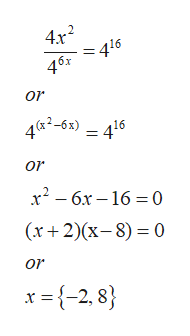# Solve the following exponential equation using properties of exponents. (Enter your answers as a comma-separated list. If an answer does not exist, enter DNE.)4x2·(1/46x)=416

Question
28 views

Solve the following exponential equation using properties of exponents. (Enter your answers as a comma-separated list. If an answer does not exist, enter DNE.)

4x2·(1/46x)=416

check_circle

Step 1

Given:

An  exponential equation

Step 2

To Determine:

Values of x such that given equation satisfied.

Step 3

Calculatio...help_outlineImage Transcriptionclose4x2 416 46x or 4 K2-63) 416 or х? — бх — 16 3D0 (х+2)(х-8) — 0 or x {-2, 8 fullscreen

### Want to see the full answer?

See Solution

#### Want to see this answer and more?

Solutions are written by subject experts who are available 24/7. Questions are typically answered within 1 hour.*

See Solution
*Response times may vary by subject and question.
Tagged in

### Exponents